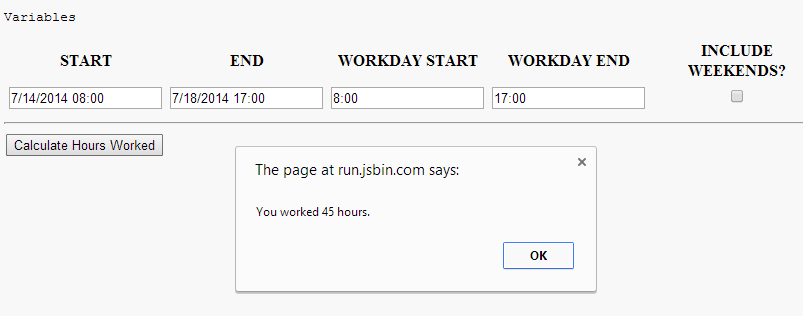Time is money, especially when that time is spent handling things like calculating hours worked and filling out time-sheets.

Recently, a user on the forums posed a question about how to go about calculating the number of hours worked between two specified dates. The request required that it must be handled through Javascript and that it must be as “exact as possible” down to the minute. So I wrote up the following quick solution and thought I would post it here on the off-chance that anyone encounters the same issue.

Let’s take a look at what information we need :

• Define the work hours during the day (e.g between 9AM and 5PM)
• Define a starting and ending date to calculate between.
• Determine if weekends are counted.

Using this information I threw together the following annotated example :

``````// Simple function that accepts two parameters and calculates
// the number of hours worked within that range
function workingHoursBetweenDates(startDate, endDate) {
// Store minutes worked
var minutesWorked = 0;

// Validate input
if (endDate < startDate) { return 0; }

// Loop from your Start to End dates (by hour)
var current = startDate;

// Define work range
var workHoursEnd = 18;
var includeWeekends = false;

// Loop while currentDate is less than end Date (by minutes)
while(current <= endDate){
// Is the current time within a work day (and if it
// occurs on a weekend or not)
if(current.getHours() >= workHoursStart && current.getHours() < workHoursEnd && (includeWeekends ? current.getDay() !== 0 && current.getDay() !== 6 : true)){
minutesWorked++;
}

// Increment current time
current.setTime(current.getTime() + 1000 * 60);
}

// Return the number of hours
return minutesWorked / 60;
}
``````

or if you would prefer to pass in all of your variables as parameters, you could use :

``````// Simple function that accepts two parameters and calculates
// the number of hours worked within that range
function workingHoursBetweenDates(startDate, endDate, dayStart, dayEnd, includeWeekends) {
// Store minutes worked
var minutesWorked = 0;

// Validate input
if (endDate < startDate) { return 0; }

// Loop from your Start to End dates (by hour)
var current = startDate;

// Define work range
var workHoursEnd = dayEnd;

// Loop while currentDate is less than end Date (by minutes)
while(current <= endDate){
// Store the current time (with minutes adjusted)
var currentTime = current.getHours() + (current.getMinutes() / 60);

// Is the current time within a work day (and if it
// occurs on a weekend or not)
if(currentTime >= workHoursStart && currentTime < workHoursEnd && (includeWeekends ? current.getDay() !== 0 && current.getDay() !== 6 : true)){
minutesWorked++;
}

// Increment current time
current.setTime(current.getTime() + 1000 * 60);
}

// Return the number of hours
return (minutesWorked / 60).toFixed(2);
}
``````

or if you want a minified version thanks to Google’s Online Closure Compiler :

``````function workingHoursBetweenDates(a,b,e,f,g){var c=0;if(b<a)return 0;for(;a<=b;){var d=a.getHours()+a.getMinutes()/60;d>=e&&d<f&&(g?  0!==a.getDay()&&6!==a.getDay():1)&&c++;a.setTime(a.getTime()+6E4)}return(c/60).toFixed(2)};
``````

Basically, it simply starts at the beginning time and iterates until the end (while monitoring the number of minutes worked during this period). By no means is this optimal, but it should serve as a very basic example of how to calculate such a value within Javascript. Please feel free to post any improvements or optimizations within the comments section as this was just a hastily thrown together solution to solve the issue at hand.

You can find a working example here :